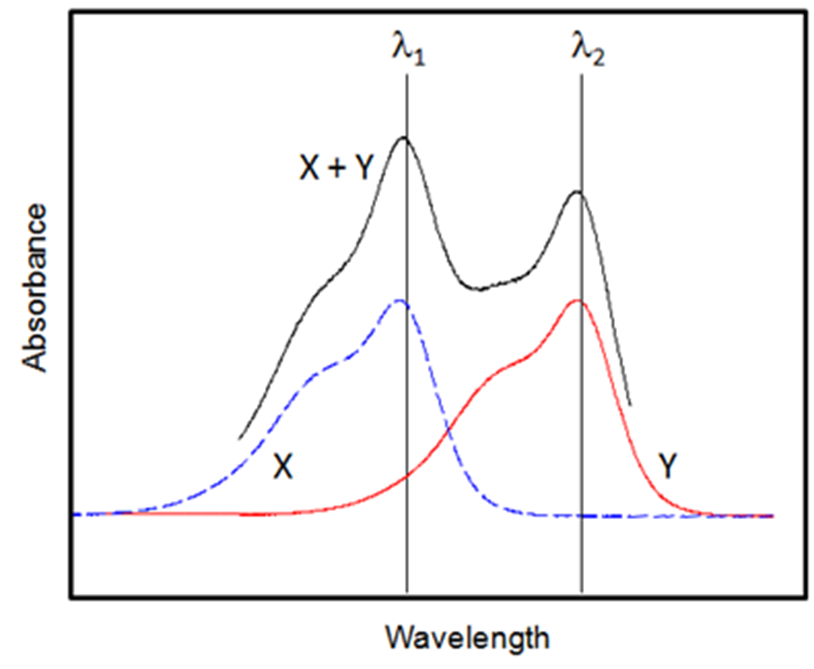# Sim4Web

Simulators for the Web (Sim4Web) is the platform that hosts our online simulations in a virtual laboratory  held in “the cloud”

## Sim4Web

Experiment 1, Identification of an Unknown Mixture

## Background

In the first part of this experiment, UV-Vis spectra will be recorded for several, simple aromatic molecules in toluene solution. Beer-Lambert plots will be constructed for each aromatic spices and the molar extinction coefficient determined. In the second part of the experiment, unknown mixtures will be investigated. Given the information derived in part one, UV-Vis spectroscopy will be used qualitatively to identify the species, and then quantitatively to determine the amount of each component present in the unknown sample.

The basis of a UV-Vis absorption meaasurement can be described by the Beer-Lambert Law which relates the incident light intensity Io at the front of a cell of length, L, (in centimetres) of a single absorbing species of concentration, c, to that which is transmitted by the sample [i.e., the intensity of light (I) which emerges from the cell]. ε is known as the molar absorption or extinction coefficient and is a measure of the probability of absorption of a photon of light by a compound.

The Beer-Lambert law is expressed formally in equation 1:

log 10 (Io/I) = – log 10 (% T/100%) = ε c L = A                       Equation 1

The ratio I/Io is known as the transmittance of the sample and is usually expressed as a percentage (%T). This is one method of describing the absorption of light by the chromophore. Clearly, from consideration of equation 1, the transmittance falls exponentially with concentration.

An alternative means of expressing the light absorbed by the sample is through the absorbance, A.

Absorbance is related to the transmittance and percentage transmittance (% T) by equation 1. Since A is also directly proportional to concentration, from equation 1, it is more convenient for quantitative analysis, to work

in terms of A rather than transmittance, see Figure 2.

Figure 2 is known as a Beer-Lambert plot and ε can be derived from the slope of the straight line. Once ε is known for a compound, it is possible

to estimate the concentration of an unknown sample by measuring A spectrophotometrically. This approach forms the basis of the procedure for determing the label content in a singly tagged polymer sample.Figure 3: The spectrum produced by a mixture of components X and Y. Analyses wavelengths for the mixture, lambda 1 and lambda 2 are also shown.

Extending this idea further, UV-Vis analysis is a powerful method for identifying and determining the concentration of unknown mixtures. This approach can be used to determine the amount of two distinct dye labels in a polymer. When there are several such components which absorb at the same wavelength their absorbances add together, so the absorbance of the sample is no longer proportional to the concentration of one component. Consider a mixture of sample X and Y as in Figure 3.

Since the total absorbance (A total ) of the solution at a given wavelength is equal to the sum of the absorbances of the individual components (as in Equation 2), it is possible to analyse the individual constituents of a mixture even when their spectra overlap:

A total = ABS 1 + ABS 2                             Equation 2

Clearly from Figure 3, there is no wavelength we can select that does not contain a contribution of absorbance from both X and Y. To overcome this problem, we must first choose wavelengths which are well separated from one another (i.e., λ1 and λ2 in Figure 3.)

The absorbance of a solution containing two chromophores, X and Y will then be equal to the sum of  the absorbances of each of the components at λ1 and λ2:

λ1 : A1 = εX1 L CX + εY1 L Cy                                      Equation 3

λ2 : A2 = εX2 L CX + εY2 L Cy                                      Equation 4

The four molar extinction (absorption) coefficients, εX1, εX2, εY1 and εY2 can be derived from standard solutions containing only X or Y, respectively. Then, if the absorbances of the mixture are measured at λ1 and λ2, the concentration of the individual components can be calculated by solving the two simultaneous equations (Equations 3 and 4).

## Experiment 1, Identification of an Unknown Mixture

In the first part of this experiment, stock solutions and dilutions will be prepared using the virtual flask module for several, simple aromatic molecules in toluene solution.

UV-Vis spectra will be recorded using the spectrometer simulator for each of the virtual samples.